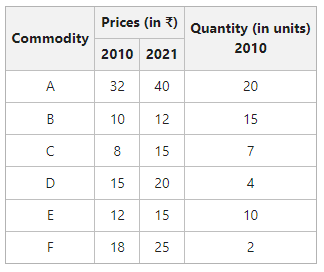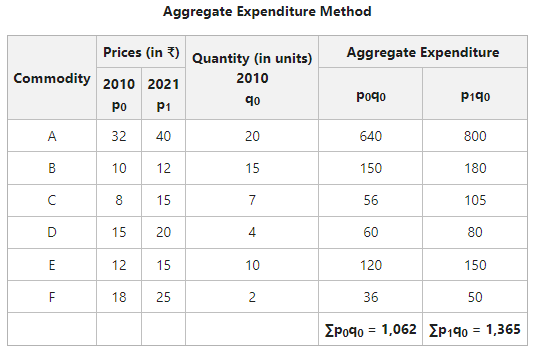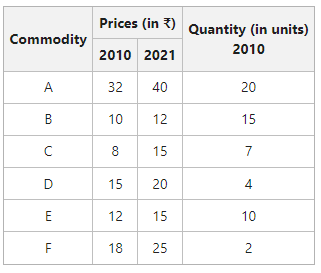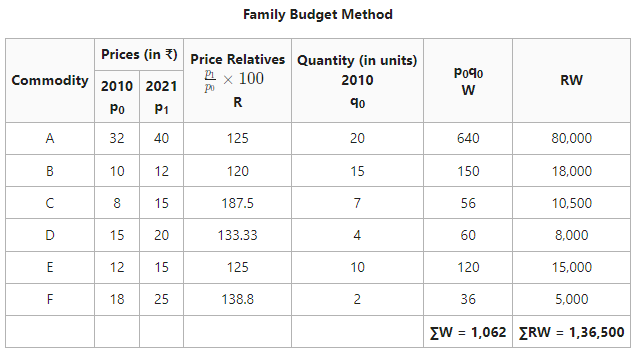GFG App
Open AppBrowser
Continue

# Methods of Constructing Consumer Price Index (CPI)

The index reflecting the average increase in the cost of the commodities consumed by a class of people and helping them maintain the same standard of living in the current year as in the base year is known as Consumer Price Index (CPI). The main aim behind their design is the measurement of the effects of change in the prices of a basket of goods and services on the purchasing power of a specific section of society during the current period with respect to the base period. Other names for Consumer Price Index are Cost of Living Index Numbers, Price of Living Index Numbers, and Retail Price Index Numbers.

### Methods of Constructing Consumer Price Index (CPI)

The two methods of constructing Consumer Price Index Numbers are Aggregate Expenditure Method or Weighted Aggregate Method, and Family Budget Method or Method of Weighted Average of Price Relatives.

#### 1. Aggregate Expenditure Method

This method is quite similar to Laspeyre’s Method of Constructing Weighted Index. For the application of this method, one has to estimate the quantities of commodities consumed by the particular group in the base year. The figures estimated are used as weights. After that, the total expenditure on each commodity for the base and current year is calculated. The steps required to determine Consumer Price Index through this method are:

Step 1: Multiply the prices of the base year (p0) with the quantities of the base year (q0) and add the resultant values to obtain the aggregate expenditure for the base year; i.e., ∑p0q0.

Step 2: Now, multiply the prices of the current year (p1) with the quantities of the base year (q0) and add the resultant values to obtain the aggregate expenditure for the base year; i.e., ∑p1q0.

Step 3: Divide the aggregate current year’s expenditure (∑p1q0) by the aggregate base year’s expenditure (∑p0q0) and multiply it by 100 to obtain the consumer price index.

Thus, the formula to determine Consumer Price Index under this method is as follows:#### Example:

Determine the cost of living index, for the following data using the Aggregate Expenditure Method.#### Solution:The Consumer Price Index of 128.53 shows that there is an increase f 28.53% in the prices in the year 2021 as compared to the year 2010.

#### 2. Family Budget Method

This method involves carefully studying the family budgets of a large number of people for whom the index is meant. After that, the aggregate expenditure of an average family on different commodities is estimated. The estimated values constitute the weights. The steps required to determine Consumer Price Index through this method are:

Step 1: First, calculate the price relatives for the current yearand denote the resultant figure by R.

Step 2: Now, multiply the price in the base year (p0) by the quantity in the base year (q0) to determine the weight of a commodity; i.e., to obtain W.

Step 3: Multiply the price relatives (R) with the weight (W) of each commodity and obtain the total; i.e., ∑RW.

Step 4: Now obtain the sum total of weights to obtain ∑W and apply the following formula:#### Example:

Determine the cost of living index for the following data using the Family Budget Method.#### Solution:The Consumer Price Index of 128.53 shows that there is an increase of 28.53% in the prices in the year 2021 as compared to the year 2010.

My Personal Notes arrow_drop_up